CBSE Sample Papers for Class 12 Chemistry Paper 2 are part of CBSE Sample Papers for Class 12 Chemistry. Here we have given CBSE Sample Papers for Class 12 Chemistry Paper 2.

## CBSE Sample Papers for Class 12 Chemistry Paper 2

 Board CBSE Class XII Subject Chemistry Sample Paper Set Paper 2 Category CBSE Sample Papers

Students who are going to appear for CBSE Class 12 Examinations are advised to practice the CBSE sample papers given here which is designed as per the latest Syllabus and marking scheme as prescribed by the CBSE is given here. Paper 2 of Solved CBSE Sample Paper for Class 12 Chemistry is given below with free PDF download solutions.

Time Allowed : 3 Hours

Max. Marks : 70

General Instructions :

• All questions are compulsory.
• Question number 1 to 5 are very short answer questions and carry 1 mark each.
• Question number 6 to 10 are short answer questions and carry 2 marks each.
• Question number 11 to 22 are also short answer questions and carry 3 marks each.
• Question number 23 is a value based question and carry 4 marks.
• Question number 24 to 26 are long answer questions and carry 5 marks each.
• Use log table, if necessary. Use of calculators is not allowed.

Questions

Question 1.
Name the non-stoichiometric point defect responsible for colour in alkali metal halides.

Question 2.
Gelatin is generally added to ice cream. Give reason.

Question 3.
Give IUPAC name of the following organic compound :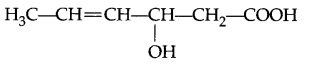Question 4.
Write the structure of Acetoxy salicylic acid.

Question 5.
Name the mono saccharides persent in lactose.

Question 6.
Differentiate between molarity and molality for a solution. How does a change in temperature influence their values?

Question 7.
A chemical reaction is of first order w.r.t. reactant A and of second order w.r.t. reactant B. How is the rate of this reaction affected when

1. the concentration of B is alone increased to three times.
2. the concentration of A as well as B are doubled?

Question 8.
Complete the following chemical equations:

1. Cu + Cone. HNO3
2. S8 + H2SO4 (conc.) →

Question 9.
Write complete chemical equations for

1. Oxidation of Fe2+ by Cr2O2-7 in acidic medium
2. Oxidation of S2O2-3 by MnO4 in neutral aqueous medium

Or
Complete the following chemical equations:

1. MnO4 (aq) + C2O2-4 (aq) + H+ (aq) →
2. Cr2O2-7 (aq) + Fe2+ (aq) + H+(aq) →

Question 10.
Which one of the following pair of sustances undergoes SN2 substitution reaction faster and why?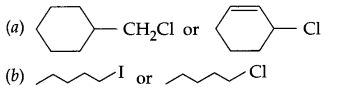Question 11.
Calcium crystallises in Face Centered Cubic structure. Radius of the atom in the metal is 141.4 pm.
(a) What is the length of the side of the unit cell?
(b) How many atoms of Calcium are there in its 5 cm3?

Question 12.
Calculate the freezing point of an aqueous solution containing 10.5 g of MgBr2 in 200 g of water (given molar mass of MgBr2 = 184 g mol-1, Kf for water = 1.86 K kg mol-1, also MgBr2 is 80% dissociated).

Question 13.
The rate of a reaction becomes four times when the temperature changes from 300 K to 320 K. Calculate the energy of activation of the reaction, assuming that it does not change with temperature. (R = 8.314 JK-1 mol-1)

Question 14.

1. How electrophoresis leads to coagulation of gold sol?
2. Explain Tyndall effect briefy by taking an example.
3. Explain why coagulation of sols will occur.p-

Question 15.
(a) Briefly explain leaching process with reaction.
(b) With the help of reactions, explain how pure Zr is obtained from impure Zr?
(c) With the help of reactions, explain how pure gold is obtained?

Question 16.
How would you carry out the following conversions? Write complete equations in each case.
(a) 1-methylcyyclohexene to 1-iodo methylcyclohexanol
(b) Styrene to 1-bromo-l-phenylethane
(c) But-4-enal to 2-Bromobutane

Question 17.

1. Why in transition elements, the melting point first increases and then decreases?
2. Why scandium (Atomic no. 21) is considered a transition metal?
3. How would you account for the following-Actinoid contraction is greater than lanthanoid contraction?

OR
With the help of chemical reactions, explain how KMnO4 is prepared from pyrolusite ore? Also, write the chemical reaction of KMnO4 with SO2 gas in acidic medium?

Question 18.
How will you bring about following conversions? Write complete equations in each case.
(a) Ethanal to 3-hydroxybutanal
(b) Benzaldehyde to benzophenone
(c) Propanone to propene

Question 19.
Give the structures of A, B and C in the following reactions: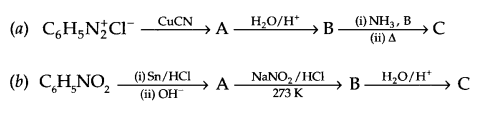Question 20.
Explain the following:
(b) Essential amino acid
(c) Pyranose Structure of β Glucose

Question 21.
Write the names and structures of the monomers of the polymers given below:
(a) Buna-S
(b) Neoprene
(c) Nylon-6,6

Question 22.

1. Give two macromolecules used as drug traget.
2. What are antiseptics?
3. What are anionic detergents?

Question 23.
Mamta, a housewife lives in Sonepat (Haryana). The tap water she gets is rich in dissolved salts. She uses ordinary washing soap for washing clothes and she finds that soaps are not very effective in cleaning her clothes. One of her neighbours, Shilpa is a student of science. Shilpa suggested to her to use synthetic detergents for washing clothes.
(i) As a science student, why did Shilpa suggest to Mamta to use synthetic detergent?
(ii) Give one chemical reaction to justify why not to use ordinary soap.
(iii) What were the values associated with the above decision?

Question 24.
What type of a battery is the lead storage battery? Write the anode and the cathode reactions and the overall reaction occurring in a lead storage battery when current is drawn from it. Calculate the emf of the following cell at 25°C:
Cu(s) | CuSO4(10-1 M)|| Ag2SO4(10-3 M) | Ag
Given Ecell° = 0.46 V
OR
(a) Write the anode and cathode reactions occurring in a commonly used mercury cell. How is the overall reaction represented?
(b) Calculate the degree of dissociation and dissociation constant of 0.01 M acetic acid at 298 K, given that
Δ0m (CH3COOH) = 39 S cm2 mol-1
Δ0m (CH3COO) = 40.9 S cm2 mol-1
Δ0m (H+) = 349.1 S cm2 mol-1

Question 25.
(a) Explain each of the following:

1. Molten PCl5 conducts electricity
2. The electron gain enthalpy of Fluorine is less than Chlorines .
3. Fluorine forms only one oxoacid, that is HOF

(b) Draw the structure of
(i) XeOF
(ii) ClF4
OR

1. Which neutral molecule would be isoelectronic with CIO?
2. Of Bi (V) and Sb(V) which may be stronger oxiding agent and why?
3. What is the basicity of H3PO3 and why?
4. Why do noble gases have very low boiling points?
5. Nitrogen is much less reactive than phosphorus at room temperature. Why?

Question 26.
(a) Arrange the following sets of compounds in increasing order according to the given instruction.

1. Phenol, 2-nitrophenol, Cyclohexanol (Acidic character)
2. pentan-l-ol, n-butane, pentanal, ethoxyethane (boiling points)

(b) Describe the mechanism of hydration of ethene to yield ethanol.
(c) Write Kolbe’s reaction with an example.
OR
(a) Explain the following name reactions with a suitable example.

1. Reimer-Tiemann reaction
2. Williamson’s synthesis

(b)

1. Haloalkanes are more soluble in organic solvent as compared to water, why?
2. Grignard’s reagent is prepared under anhydrous conditions, why?
3. Phenol is more acidic than ethanol, why?

F-centre or metal excess defect

Gelatin acts as an emulsifying agent as well as prevents crystallisation of water.

3-Hydroxyhex-4-enoic acid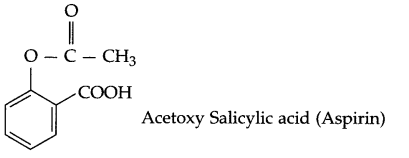β-D-galactose and β-D-glucose.

• Molarity : It is the number of moles of solute per litre of solution.
• Molality : It is number of moles of solute per kilogram of solvent.

In case of molarity, its value will decrease with increasing temperature but molality remains unchanged with change in temperature.

According to given information the rate law equation is
rate = k[A] [B]2

1. On increasing [B] three times
rate1 = k [A] [3B]2
= 9 k[A] [B]2
i.e. rate becomes nine times.
2. When [A] and [B] gets doubled, then
rate2 = K[2A] [2B]2 = 8 K[A] [B]2
i.e. rate becomes 8 times.

1. Cu + 4HNO3 (conc.) → Cu (NO3)2 + 2NO2 + 2H2O
2. S8 + 16H2SO4 (conc.) → 24SO2 + 16H2O

(a) Cr2O2-7 + 6Fe2+ + 14+ → 2Cr3+ + 6Fe3+ + 7H2O
(b) 8MnO4 + 3S2O2-3 + H2O → 8MnO2 + 6SO2-4 + 2OH
OR
(a) 2MnO4 + 5S2O2-4 + 16H+ → 2Mn2+ + 8H2O + 10CO2
(b) CrO2-7 + 3H2S + 8H+ → 2Cr3+ + 3S + 7H2OSince calcium crystallises in fee structure, soFrom questionSince K2 = 4K1
T1 = 300 K, T2 = 320 K, R = 8.314 JK-1 mol.
from Arrhenius relation:1. The colloidal particles move towards oppositely charged electrode i.e. electropheoresis takes place and it will lead to coagulation of gold sol.
2. The path of light becomes visible due to scattering of light by particles of starch sol and tyndall effect will take place.
3. Coagulation of sol will occur due to neutralisation of charges on the colloidal particles.

(a) The process of leaching with NaOH (aq) is used for leaching of bauxite ore to get pure alumina (Al2O3). The reaction involved is
Al2O3(g) + 2NaOH(aq) + 3H2O(g) → Al2O32CH2O↓ + 2NaHCO3 (aq)
Precipitate is filtered, washed and dried, followed by heating to get pure Al2O3.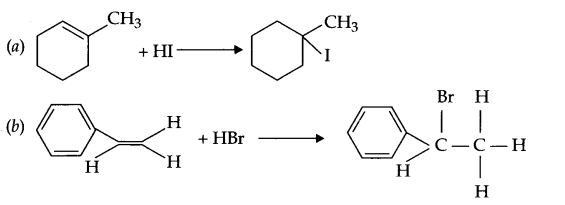1. It is due to strong metal metal bond which is there because of small size and large number of unpaired electrons in the valence shell.
2. Sc (21) → [Ar] 3d14s2
It contains partially filled d-orbital in the ground state, i.e. 3d1 configuration, hence it is d-orbital considered as a transition metal.
3. It is due to poorer shielding of 5f electrons in actinoids as compared to 4f electrons of lanthanoids.

OR
Preparation of KMnO4 from pyrolusite ore :Reaction with SO2
2MnO4 + 5SO2 + 2H2O $$\underrightarrow { { H }^{ + } }$$ 2Mn2+ + 5SO2-4 + 4H+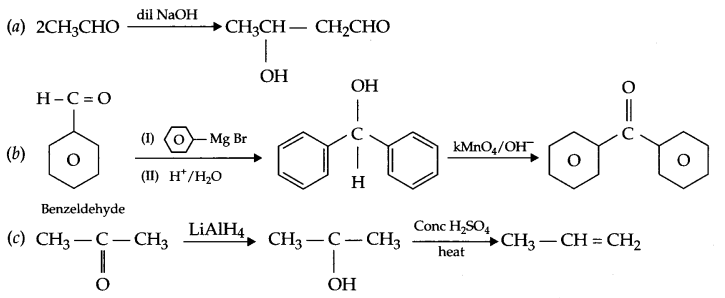Answer 19.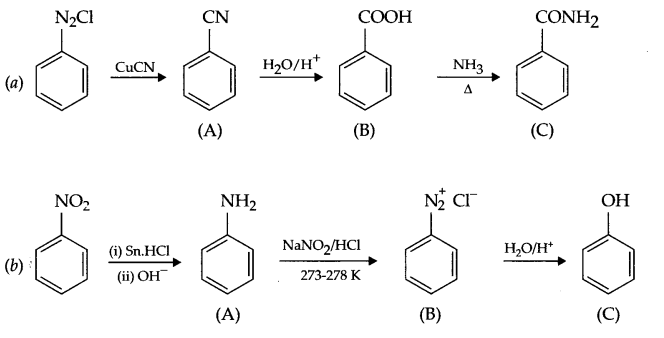(a) Peptide linkage : Proteins are connected to each other through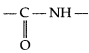(amide linkage) bond which is called peptide bond or linkage in protein chemistry,
e.g.(b) Essential amino acid : The amino acids which must be taken in diet regularly are known as essential amino acids.
(c) Pyranose structure of β-glucose is as follows: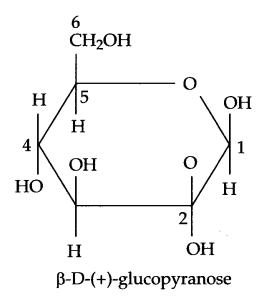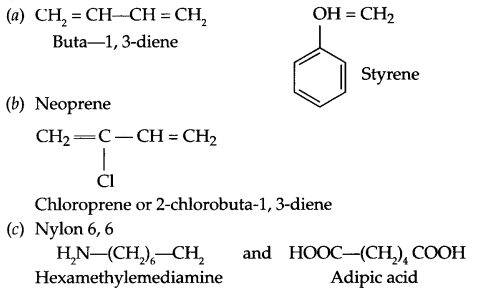1. Protein, enzymes, hormones etc.
2. Antiseptics : The class of compounds used to kill microorganisms on living cells are known as antiseptics.
3. These are sodium salts of sulphonated long chain alcohols or hydrocarbons, since large part of such detergent is anionic and cleansing action is also due to this part. Hence such detergents are known as anionic detergents. Most of the household detergents are anionic.

(a) Synthetic detergents provide cleaning in soft water as well as in hard water.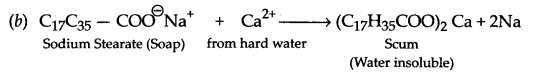(c) Values associated are knowledge of chemistry, concern for friend, cleanliness and environment protection, (any two)

It is a secondary battery, i.e. rechargeable
Cell reaction during battery in use:
At anode : Pb(s) + SO2-4(aq) → PbSO4(aq) + 2e
Cathode : Pb(s) + SO2-4(aq) + 4H+(aq) + 2e → PbSO4(s) + 2H2O (l)
Net reaction : Pb(s) + PbO2(s) + 2H2SO4(aq) → 2PbSO4 + 2H2O(l)

(b) Cell reactions at
Anode → Cu(s) Cu2+ + x  Here, n = 2
Cathode → (Ag+ + e → Ag) × 2
Net reaction :
Cu(s) + 2Ag+ → Cu2+ + 2Ag
At 298 K, Nemst equation for emf of cell: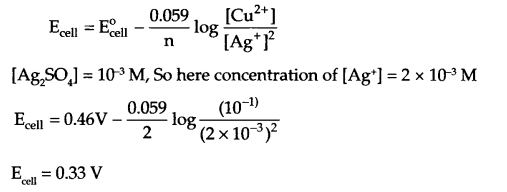OR
Reaction of mercury cell at
Anode : Zn (Hg) + 20H → ZnO(s) + H2O + 2e
Cathode : HgO + 2H2O + 2e → Hg(l) + 2OH
Overall reaction:
Zn(Hg) + HgO(s) → ZnO(s) + Hg(s)
[since no ion is involved during the overall reaction, so cell potential remains constant during the entire life of the cell]

(b) from question (Δ0m) = 39 S cm2 mol-1
limiting molar conductivity (Δ0m) for acetic acid
Δ0m (CH3COOH) = λCH3COO + λ°H+
= 349.1 + 40.9 – 390 S cm2 mol-1
Now, Degree of dissociation,α = 0.1
Dissociation constant (Ka) for fa acetic acid :
K = Cα2 = (0.01) (0.1)2 = 10-4

(a)

1. In molten state PCl5 exist as [PCl4]+ and [PCl6] ions, hence it conducts electricity.
2. It is due to high electron density in valence shell of fluorine.
3. Due to absence of d orbitals in F, it cannot expand its covalency.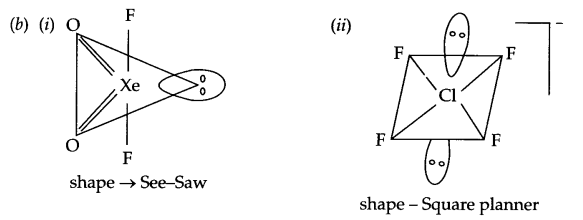OR

1. OF2 or ClF
2. Bi(V) is an stronger oxidising agent, reason being Bi(III) is more stable than Bi(V) because of inert pair effect.
3. Structure of H3PO3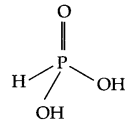Its basicity is two and the reason being is, it consists of two OH groups, hence only 2H+ ions can be released.
4. Noble gases are monotomic gas molecules and hence only weak dispersion forces are there. As a result they have low boiling point.
5. Triple bond present between two nitrogen atoms is much stronger than P-P single bond, hence nitrogen is less reactive than phosphorous.

(a)

1. Cyclohexanol < phenol < 2-nitrophenol
2. n-butane < ethoxyethane < pentanal < pentan-1-ol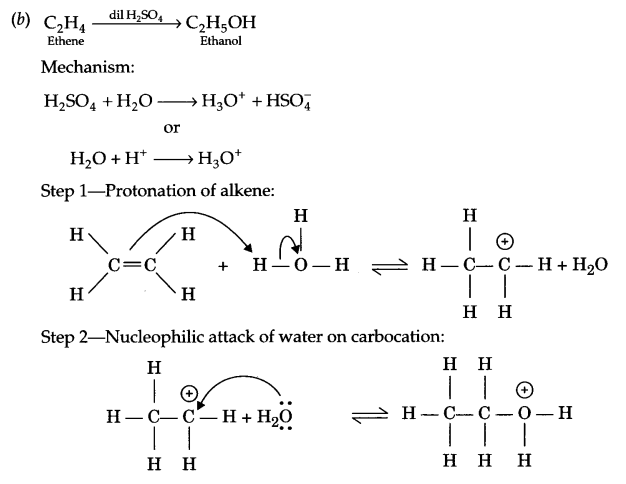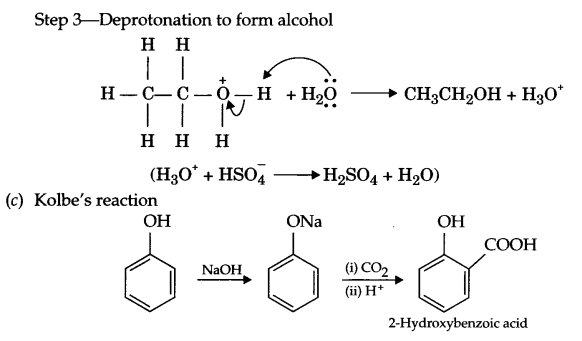OR
(a)

1. Reimer-Tiemann reaction:2. Williamson’s synthesis:
An alkyl halide reacts with sodium alkoxide to form ether.
R-X + R’- ONa → R-O-R’ + NaX
CH3Cl + NaOC2H5 → CH3-O-CH2-CH3 + NaCl

(b)

1. Haloalkanes are unable to form hydrogen bond with water but bonding of haloalkanes with organic solvent is as strong as among haloalkanes. Due to this reason haloalkanes are more soluble in organic solvent than water.
2. Grignard reagent reacts immediately with water to form alkane, hence they are prepared under anhydrous conditions.
RMgX + H2O → RH + MgX(OH)
3. Phenoxide ion is resonance stablised, hence phenol is more acidic than ethanol (ethoxide ion is destablised by +I effect of alkyl group).

We hope the CBSE Sample Papers for Class 12 Chemistry Paper 2 help you. If you have any query regarding CBSE Sample Papers for Class 12 Chemistry Paper 2, drop a comment below and we will get back to you at the earliest.